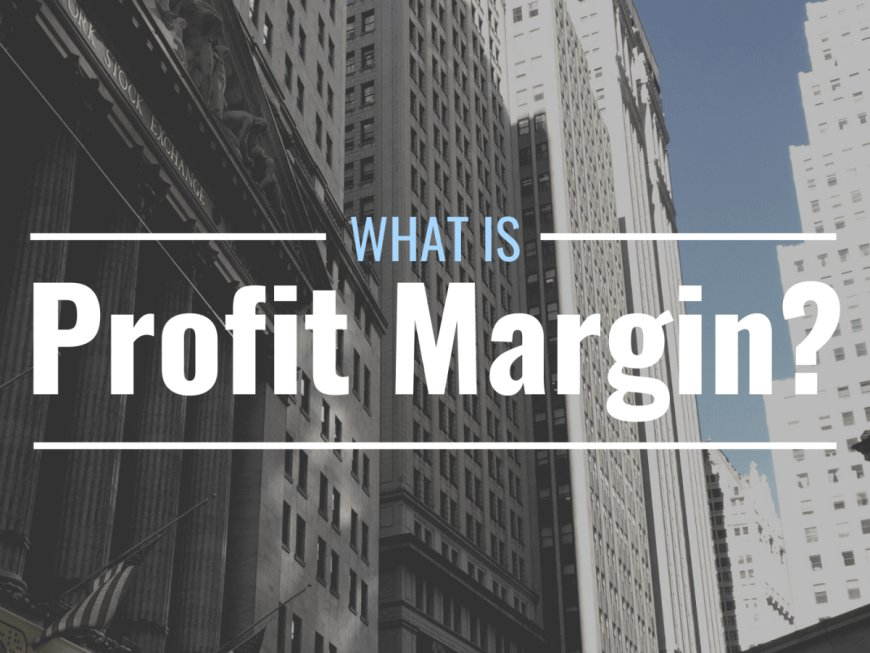# Introduction to Profit Margin for Kids & Adults

Jul 15, 2023 - 11:23
0  5PROFIT MARGIN:

Profit margin is a way to understand how much money a business makes from selling things. It helps us figure out the percentage of profit a company earns from its sales.

Profit margin helps businesses understand how well they are doing financially. A higher profit margin means the business is making more money from its sales, while a lower profit margin means it is making less. It is important for businesses to have a healthy profit margin so they can cover their costs and make a profit.

Remember, profit margin tells you the percentage of profit a business earns from its sales. It helps businesses and individuals understand how much money they are making compared to what they are selling.

The formula for calculating profit margin is:

Profit Margin = (Profit / Selling Price) * 100

To calculate the profit margin, you need to know the profit (which is the money you make after subtracting the cost from the selling price) and the selling price (which is the price at which you sell your product or service).

Let's break it down step by step:

1. Calculate the profit by subtracting the cost from the selling price. Profit = Selling Price - Cost.

1. Divide the profit by the selling price. This gives you a decimal value.

1. Multiply the decimal value by 100 to convert it into a percentage. This gives you the profit margin.

Examples for Kids:

You sell each glass of lemonade for ₹10, and it costs you ₹5 to make one glass (including lemons, sugar, and cups). To find the profit, subtract the cost (₹5) from the selling price (₹10), which gives us a profit of ₹5. The profit margin is 50% because the profit (₹5) is half of the selling price (₹10).

1. Candy Sales:

You buy a bag of candies for ₹20 and sell each candy for ₹5. If you sell all the candies in the bag, you will earn ₹100. The profit is ₹80 because you subtract the cost (₹20) from the total earnings (₹100). The profit margin is 400% because the profit (₹80) is four times the cost (₹20).

1. Toy Store:

You buy a toy for ₹500 and sell it for ₹800. The profit is ₹300 because you subtract the cost (₹500) from the selling price (₹800). The profit margin is 60% because the profit (₹300) is 60% of the selling price (₹800).

1. Artwork Sales:

You create a painting and sell it for ₹1,000. The cost of the materials used is ₹200. Your profit is ₹800 because you subtract the cost (₹200) from the selling price (₹1,000). The profit margin is 80% because the profit (₹800) is 80% of the selling price (₹1,000).

1. Vegetable Garden:

You grow vegetables in your garden and sell them at a local market. Let's say you sell a basket of vegetables for ₹200, and it costs you ₹100 to grow them. Your profit is ₹100, and the profit margin is 50% because the profit (₹100) is half of the selling price (₹200).

1. Clothing Store:

You buy a shirt for ₹500 and sell it for ₹1,000. The profit is ₹500 because you subtract the cost (₹500) from the selling price (₹1,000). The profit margin is 50% because the profit (₹500) is 50% of the selling price (₹1,000).

1. Restaurant:

You sell a meal for ₹500, and it costs you ₹300 to prepare the ingredients and pay the staff. Your profit is ₹200 because you subtract the cost (₹300) from the selling price (₹500). The profit margin is 40% because the profit (₹200) is 40% of the selling price (₹500).

1. Electronics Store:

You buy a phone for ₹10,000 and sell it for ₹15,000. The profit is ₹5,000 because you subtract the cost (₹10,000) from the selling price (₹15,000). The profit margin is 33.33% because the profit (₹5,000) is 33.33% of the selling price (₹15,000).

1. Bakery:

You sell a cake for ₹500, and it costs you ₹200 to make it. Your profit is ₹300 because you subtract the cost (₹200) from the selling price (₹500). The profit margin is 60% because the profit (₹300) is 60% of the selling price (₹500).

1. Online Store:

You buy a product for ₹1,000 from a wholesaler and sell it for ₹2,000 on your website. The profit is ₹1,000 because you subtract the cost (₹1,000) from the selling price (₹2,000). The profit margin is 50% because the profit (₹1,000) is 50% of the selling price (₹2,000).

Profit margin is an important measure that shows how much profit a business is making from its sales. It helps businesses understand if they are earning enough money to cover their expenses and make a profit. A higher profit margin means the business is doing well and making more money. However, a lower profit margin might indicate that the business needs to find ways to increase revenue or reduce costs to improve profitability. Profit margin is like looking at the money you make compared to the money you spend, and it helps businesses and individuals understand how well they are doing financially.

### Importance of HR in Banking